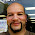## Wednesday, October 2, 2013

### HP Prime Program: Sampling Without Replacement

SAMPLE - Generate a list of random integers from 1 to N using sample without replacement.

Author: Eddie Shore
Date: 10/1/2013

Syntax: SAMPLE(L, N)
L = length of desired list
N = high integer
If L > N, an error occurs.

Output: random sample

Program:
EXPORT SAMPLE(L,N)
BEGIN
// length, number
LOCAL I, K, T, num;

// error cond
IF L > N THEN
1/0;
END;

// main program
L1:=MAKELIST(0,X,1,L,1);
L1(1):=RANDINT(N-1)+1;
I:= 2;

REPEAT
num:=RANDINT(N-1)+1;
T:=1;

// test for uniqueness
FOR K FROM 1 TO I DO
IF num == L1(K) THEN
T:=T + 1;
END;
END;

IF T == 1 THEN
L1(I) := num;
I := I + 1;
END;

UNTIL I == L + 1;
RETURN L1;
END;

Examples:
SAMPLE(5,9) (length of 5, n = 9) can generate:
{5, 4, 8, 2, 6}
{9, 7, 8, 1, 2}
{4, 3, 6, 5, 2}

This blog is property of Edward Shore. 2013

1.Great work and very useful example. What is the standard file extension for Prime programs? Want to add that to my editor to syntax color them.

1.Thank you. I am not sure if Prime programs have an extension. I have to use Notepad to copy programs and save the copies as UTC-8 encoded files.

2.Actually it is .hpprgm

1.Thanks Pedro!

Eddie

3.Hi Edward,
FYI you have a tiny error in the above program:
T:=1
T:=1;
(i.e. the semicolon at the end of the line is missing).

Thank you for posting example programs up on your blog. It's very helpful to help see example code when learning a [new version of a] programming language.

Also, in the spirit of sharing (for other budding programmers out there), here's my version of the same program -- the only difference being that you can specify whether to generate a unique list of random numbers or not.

REPEATEDNUMGENERATED();
EXPORT RandomNumGen(TotListSize,BiggestInt,UniqueNumListTrueFalse)
BEGIN

LOCAL ListRowPos, RandomGeneratedNum, ErrorMsg;

//check for infinite loop situation
if UniqueNumListTrueFalse == 1 and TotListSize > BiggestInt then
ErrorMsg:= "Unique numbers in list requested. Ensure the biggest integer allowed is at least as large as the requested list size.";
msgbox(ErrorMsg);
RETURN ErrorMsg;
end;

//dimension L1 array to cater for TotListSize
L1:= MAKELIST(0,X,1,TotListSize,1);
//populate first row of TotListSize
L1(1):= RANDINT(BiggestInt-1)+1;

//populate rest of array (row 2 onwards)
for ListRowPos from 2 to TotListSize do
RandomGeneratedNum:= RANDINT(BiggestInt-1)+1;
if UniqueNumListTrueFalse == 1 then
repeat
RandomGeneratedNum:= RANDINT(BiggestInt-1)+1;
until REPEATEDNUMGENERATED(RandomGeneratedNum,(ListRowPos-1)) == 0;
end;
L1(ListRowPos):= RandomGeneratedNum;

end;

END;

//subroutine called
REPEATEDNUMGENERATED(NumberInQuestion,FilledArraySoFar)
BEGIN
LOCAL FilledListRowPos, RepeatedTrueFalse:=0;

for FilledListRowPos from 1 to FilledArraySoFar do
if L1(FilledListRowPos) == NumberInQuestion then
RepeatedTrueFalse:= 1;
end;
end;

RETURN RepeatedTrueFalse;
END;

1.Tonto,

The blog has been corrected. I appreciate you pointing out my typo. Much appreciated.

Eddie

### TI-84 Plus CE: Simple Elliptic Curves Determined by Two Points

TI-84 Plus CE:  Simple Elliptic Curves Determined by Two Points Introduction The program ECURVE determines the coefficients of a simple...